Vectors - Motion and Forces in Two Dimensions - Lesson 1 - Vectors: Fundamentals and Operations

# Vectors and Direction

A study of motion will involve the introduction of a variety of quantities that are used to describe the physical world. Examples of such quantities include distance, displacement, speed, velocity, acceleration, force, mass, momentum, energy, work, power, etc. All these quantities can by divided into two categories - vectors and scalars. A vector quantity is a quantity that is fully described by both magnitude and direction. On the other hand, a scalar quantity is a quantity that is fully described by its magnitude. The emphasis of this unit is to understand some fundamentals about vectors and to apply the fundamentals in order to understand motion and forces that occur in two dimensions.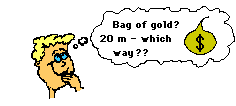Examples of vector quantities that have been previously discussed include displacement, velocity, acceleration, and force. Each of these quantities are unique in that a full description of the quantity demands that both a magnitude and a direction are listed. For example, suppose your teacher tells you "A bag of gold is located outside the classroom. To find it, displace yourself 20 meters." This statement may provide yourself enough information to pique your interest; yet, there is not enough information included in the statement to find the bag of gold. The displacement required to find the bag of gold has not been fully described. On the other hand, suppose your teacher tells you "A bag of gold is located outside the classroom. To find it, displace yourself from the center of the classroom door 20 meters in a direction 30 degrees to the west of north." This statement now provides a complete description of the displacement vector - it lists both magnitude (20 meters) and direction (30 degrees to the west of north) relative to a reference or starting position (the center of the classroom door). Vector quantities are not fully described unless both magnitude and direction are listed.

###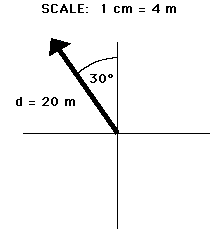Representing Vectors

Vector quantities are often represented by scaled vector diagrams. Vector diagrams depict a vector by use of an arrow drawn to scale in a specific direction. Vector diagrams were introduced and used in earlier units to depict the forces acting upon an object. Such diagrams are commonly called as free-body diagrams. An example of a scaled vector diagram is shown in the diagram at the right. The vector diagram depicts a displacement vector. Observe that there are several characteristics of this diagram that make it an appropriately drawn vector diagram.

• a scale is clearly listed
• a vector arrow (with arrowhead) is drawn in a specified direction. The vector arrow has a head and a tail.
• the magnitude and direction of the vector is clearly labeled. In this case, the diagram shows the magnitude is 20 m and the direction is (30 degrees West of North).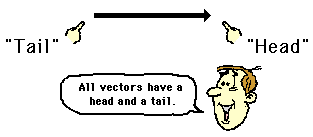### Conventions for Describing Directions of Vectors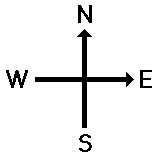Vectors can be directed due East, due West, due South, and due North. But some vectors are directed northeast (at a 45 degree angle); and some vectors are even directed northeast, yet more north than east. Thus, there is a clear need for some form of a convention for identifying the direction of a vector that is not due East, due West, due South, or due North. There are a variety of conventions for describing the direction of any vector. The two conventions that will be discussed and used in this unit are described below:

1. The direction of a vector is often expressed as an angle of rotation of the vector about its "tail" from east, west, north, or south. For example, a vector can be said to have a direction of 40 degrees North of West (meaning a vector pointing West has been rotated 40 degrees towards the northerly direction) of 65 degrees East of South (meaning a vector pointing South has been rotated 65 degrees towards the easterly direction).
2. The direction of a vector is often expressed as a counterclockwise angle of rotation of the vector about its "tail" from due East. Using this convention, a vector with a direction of 30 degrees is a vector that has been rotated 30 degrees in a counterclockwise direction relative to due east. A vector with a direction of 160 degrees is a vector that has been rotated 160 degrees in a counterclockwise direction relative to due east. A vector with a direction of 270 degrees is a vector that has been rotated 270 degrees in a counterclockwise direction relative to due east. This is one of the most common conventions for the direction of a vector and will be utilized throughout this unit.

Two illustrations of the second convention (discussed above) for identifying the direction of a vector are shown below.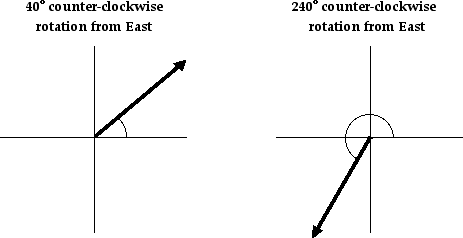Observe in the first example that the vector is said to have a direction of 40 degrees. You can think of this direction as follows: suppose a vector pointing East had its tail pinned down and then the vector was rotated an angle of 40 degrees in the counterclockwise direction. Observe in the second example that the vector is said to have a direction of 240 degrees. This means that the tail of the vector was pinned down and the vector was rotated an angle of 240 degrees in the counterclockwise direction beginning from due east. A rotation of 240 degrees is equivalent to rotating the vector through two quadrants (180 degrees) and then an additional 60 degrees into the third quadrant.###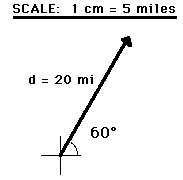Representing the Magnitude of a Vector

The magnitude of a vector in a scaled vector diagram is depicted by the length of the arrow. The arrow is drawn a precise length in accordance with a chosen scale. For example, the diagram at the right shows a vector with a magnitude of 20 miles. Since the scale used for constructing the diagram is 1 cm = 5 miles, the vector arrow is drawn with a length of 4 cm. That is, 4 cm x (5 miles/1 cm) = 20 miles.

Using the same scale (1 cm = 5 miles), a displacement vector that is 15 miles will be represented by a vector arrow that is 3 cm in length. Similarly, a 25-mile displacement vector is represented by a 5-cm long vector arrow. And finally, an 18-mile displacement vector is represented by a 3.6-cm long arrow. See the examples shown below.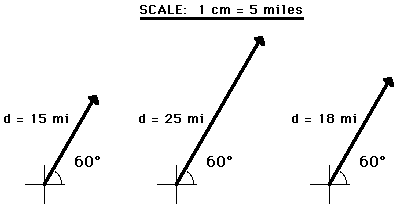In conclusion, vectors can be represented by use of a scaled vector diagram. On such a diagram, a vector arrow is drawn to represent the vector. The arrow has an obvious tail and arrowhead. The magnitude of a vector is represented by the length of the arrow. A scale is indicated (such as, 1 cm = 5 miles) and the arrow is drawn the proper length according to the chosen scale. The arrow points in the precise direction. Directions are described by the use of some convention. The most common convention is that the direction of a vector is the counterclockwise angle of rotation which that vector makes with respect to due East.

In the remainder of this lesson, in the entire unit, and in future units, scaled vector diagrams and the above convention for the direction of a vector will be frequently used to describe motion and solve problems concerning motion. For this reason, it is critical that you have a comfortable understanding of the means of representing and describing vector quantities. Some practice problems are available on site at the following web page:

Next Section: